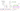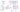## Matrix Multiplication

#### Matrix-Vector Multiplication

We map the column of the vector onto each row of the matrix, multiplying each element and summing the result.

$\begin{bmatrix} a & b \\ c & d \\ e & f \end{bmatrix} * \begin{bmatrix} x \\ y \end{bmatrix} = \begin{bmatrix} a*x + b*y \\ c*x + d*y \\ e*x + f*y\end{bmatrix}$

The result is a vector. The vector must be the second term of the multiplication. The number of columns of the matrix must equal the number of rows of the vector.

An m x n matrix multiplied by an n x 1 vector results in an m x 1 vector.

Efficient way of doing a large number of predictions for a given hypothesis function:#### Matrix-Matrix Multiplication

We multiply two matrices by breaking it into several vector multiplications and concatenating the result.

$\begin{bmatrix} a & b \\ c & d \\ e & f \end{bmatrix} * \begin{bmatrix} w & x \\ y & z \end{bmatrix} = \begin{bmatrix} a*w + b*y & a*x + b*z \\ c*w + d*y & c*x + d*z \\ e*w + f*y & e*x + f*z\end{bmatrix}$

An m x n matrix multiplied by an n x o matrix results in an m x o matrix. In the above example, a 3 x 2 matrix times a 2 x 2 matrix resulted in a 3 x 2 matrix.

To multiply two matrices, the number of columns of the first matrix must equal the number of rows of the second matrix.

Efficient way of doing a large number of predictions for more than one competing hypothesis functions:#### Matrix Multiplication Properties

• Matrices are not commutative: $A * B \neq B * A$
• Matrices are associative: $(A * B) * C = A * (B * C)$